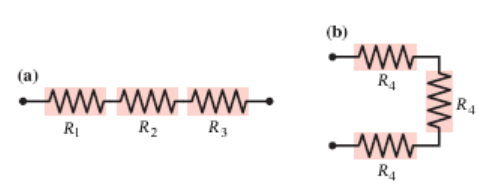# Problem: What is the equivalent resistance of each group of resistors shown in the figure below? In the figure, R1 = 2.0 ?, R2 = 3.5?, R3 = 7.5 ?, and R4 = 2.0 ?.(a) ______ ?(b) ______ ?

###### FREE Expert Solution

Equivalent resistance for series capacitors:

$\overline{){{\mathbf{R}}}_{\mathbf{e}\mathbf{q}}{\mathbf{=}}{{\mathbf{R}}}_{{\mathbf{1}}}{\mathbf{+}}{{\mathbf{R}}}_{{\mathbf{2}}}{\mathbf{+}}{{\mathbf{R}}}_{{\mathbf{3}}}}$

(a)

The resistors are in series:

88% (374 ratings)###### Problem Details

What is the equivalent resistance of each group of resistors shown in the figure below? In the figure, R1 = 2.0 ?, R2 = 3.5?, R3 = 7.5 ?, and R4 = 2.0 ?.

(a) ______ ?

(b) ______ ?Frequently Asked Questions

What scientific concept do you need to know in order to solve this problem?

Our tutors have indicated that to solve this problem you will need to apply the Combining Resistors in Series & Parallel concept. You can view video lessons to learn Combining Resistors in Series & Parallel. Or if you need more Combining Resistors in Series & Parallel practice, you can also practice Combining Resistors in Series & Parallel practice problems.